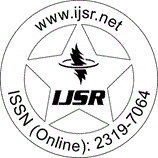International Journal of Science and Research (IJSR)
Call for Papers | Fully Refereed | Open Access | Double Blind Peer Reviewed

Research Paper | Statistics | Nigeria | Volume 8 Issue 11, November 2019

# Generalised Model of Optimum Stratification Allocation Size and Variance for Linear and Non-Linear Cost Function Considering Other Allocation Procedures

Abstract: In this paper, consideration is given to a generalised model for optimum allocation of multivariate sampling with linear and nonlinear cost function given that there is only one varying cost, the problem of determining the optimum allocation are formulated as a generalized programming problem and solved using Lagrange multiplier technique to minimize the variance subject to a given cost. A generalized sample size and variance is derived and other factors like when the cost is constant, when the sample size for a given cost is not known, when the sample size for a specified variance is not known and when a proportional allocation or equal allocation is required for a given cost and a proportionality constant is considered. Data from the sample frame of the final result of 1991 census of Owo local government area of Ondo State which encompasses of 117 localities is extracted from the National Population Commission (NPC), its inhabitants is used as character of study for the purpose of numerical illustration.

Keywords: Optimum allocation, sample size, cost function

Edition: Volume 8 Issue 11, November 2019,

Pages: 1406 - 1411